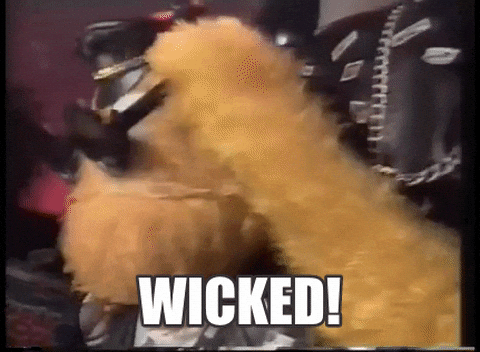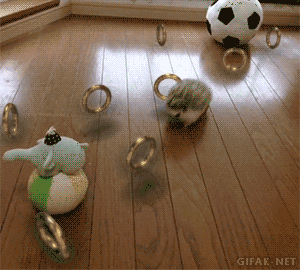# Monoids deployed

• swift
• fp

First of all, an explanation of the title is in order. I used the word “deployed” just because it kind of rhymed with “Monoid”. It’s probably a better choice than what I was originally going to use; “Monoids on Steroids”.

Anyways, this post will be an attempt to explain what monoids are, why they are useful and most importantly, how they can be used. We will also build a simple shapes library in Haskell and Swift as an example.

To start off with, here is an example of a monoid. Ready?

``````1 + 0 = 1
0 + 1 = 1
1 + (2 + 3) = 6
(1 + 2) + 3 = 6
``````

If you understand these highly complex mathematical equations, you understand what monoids are. Congratulations 🎉🎉🎉.

# Formal Definition

## Mathematical

A monoid is a tuple `(M, op, e)` where

• M is a set of element
• `op` is an associative binary operation on two elements `M`, returning a new element of `M`
• `e` is an element of `M`, neutral for `op` on both left and right side

So for example, the above addition operator would form the following tuple of `(Int, +, 0)`

## Statically typed languages

Since we are programmers, let us translate the above definition to code. A monoid consists of a type `T` and a function `f` where

## `f` takes an instance of `T` and returns an instance of `T`

`func foobar(_ in: String, _in2: String) -> String`

The above statement says that a binary operation of monoids takes two elements of type `T` and will always return `T`. The input type is same as the output type. This property is very important as it will allows us to chain operations indefinitely.

## `f` is associative

Associative property is when `f(c, f(a, b)) == f(f(c, a), b)` holds true.

In other words, we are looking for the situation where something like `1 + (2 + 3) == (1 + 2) + 3` makes sense. This allows us to not worry about the ordering when we compose functions. We will see examples of this later.

## `f` has a neutral element `e` where `f(e, a) == f(a, e)`

We are looking for `e` that allows `foobar(NEUTRAL_ELEMENT, 5) == foobar(5, NEUTRAL_ELEMENT)`

For example, `0` is the neutral element for the addition operator since `0 + 3 == 3 + 0`

# Monoids everywhere

By now, you hopefully have a sense of what monoids are. And the funny thing is, you probably have been using monoids all along without evening knowing it. Here are some examples of commonly used monoids that are unfortuantley not acknowledged as monoids most of the time.

• Integers under addition with neutral element zero
• Integers under multiplication with neutral element one
• Sequential containers under concatenation (`String`, `List`)
• Associative containers under union (`map`, `set`)

# But why should I care?

Th idea of monoids come from the realms of functional programming where the ultimate goal is to come up with simple abstractions that can later be composed to create complex behaviors. Monoids are a great representation of the FP mindset as it gives us a way to build complexity out of simplicity. This means that before you dive into a problem, you can start by formulating a very simple idea. Once you solidify this idea, you can then use it to build complex objects that will eventually solve your problem.

# ASCII Art Generator

To see how monoids can be used, we will create a library with power enough abstractions that will allows us to create some interesting ASCII art. Our library will mainly deal with creating geometric shapes.1 As we go through the code, I will demonstrate each example in Haskell and then follow up with Swift.

## Abstracting a “Shape”

Here, we will define a shape as a function that takes a coordinate point as an input and returns a boolean as an output. The idea is simple; you ask the function if the point `(x, y)` is contained in the shape and, if `true` is returned, we then know it’s part of the shape.

To define a shape, we will make a `Shape` type with the function - `isInShape` - I just described above. We will also define some typealiases to make life easier. Notice that we are using a generic struct. This is so that we can use not only `Coord2D` but also `Coord3D`, `Coord4D` in the future.

``````newtype Shape coord = Shape {
isInShape :: coord -> Bool
}
type Coord2D = (Double, Double)
type Shape2D = Shape Coord2D
``````
``````struct Shape<C> {
let isInShape : (C) -> (Bool)
}
typealias Coord2D = (Double, Double)
typealias Shape2D = Shape<Coord2D>
``````

Because we are describing shapes as functions, we can easily define the complement of a shape. For example, we can make the function `outside`. All it would have to do is negate the predicate of the input shape.

``````outside :: Shape coord -> Shape coord
outside s = Shape (not . isInShape s)
``````
``````// Thanks to u/thisischemistry, code is now more understandable and self-documenting
extension Shape {
func outside() -> Shape {
let notInOriginalShape = { coord in
!(self.isInShape(coord))
}
return Shape(isInShape: notInOriginalShape)
}
}
``````

## Creating Shapes

To define a shape, we need to specify the `isInShape` function to describe whether a point belongs in the shape or not. Here’s how we would define a disk.

``````disk :: Coord2D -> Radius -> Shape2D
Shape \$ \coord -> euclidianDistance center coord <= radius
``````
``````extension Shape {
isInShape = { coord in
guard let coord = coord as? Coord2D else { fatalError("Center must be a 2D point") }
return euclidianDistance(p1: center, p2: coord) < radius
}
}
}
``````

Now, we can experiment and have some fun with some sample coordinates

``````let disk = Shape<Coord2D>(center: (10.0, 10.0), radius: 8.00)
disk.isInShape((13.0, 13.0))
> true
``````

And with a simple loop, we can print the disk out. The output looks a bit off because the line widths are larger than the character spacings. But hey, still pretty cool, eh?

``````      *******
***********
*************
*************
***************
***************
***************
***************
***************
***************
***************
*************
*************
***********
*******
``````## Complex shapes

Now that we have our basic idea down, let’s stat complicating things. And since we are in the realm of FP, what would this post be without some composition!? Let’s try to build cooler shapes via composition.

We can first start by defining the `intersect` function which defines a new shape within the region of intersection.

``````intersect :: Shape coord -> Shape coord -> Shape coord
intersect s1 s2 =
Shape \$ \coord -> isInShape s1 coord && isInShape s2 coord
``````
``````extension Shape {
func intersect(_ s1: Shape) -> Shape {
return Shape { coord in
self.isInShape(coord) && s1.isInShape(coord)
}
}

let disk = Shape<Coord2D>(center: (10.0, 10.0), radius: 8.00)
let square = Shape<Coord2D>(origin: (7.0, 7.0), width: 6, height: 6)
let ring = disk.intersect(square.outside())
``````

Printing `ring` yields the below result

``````      ********
***********
*************
*************
****       ****
****       ****
****       ****
****       ****
****       ****
****       ****
****       ****
*************
*************
***********
*******
``````

Kind of looks like those rings from Sonic, eh?# Wrapping Up

I hope the shapes example demonstrated how great monoids are when it comes to composition and abstraction. Personally, I have constantly failed to recognize the fact that I have been using something people have discovered, named and implemented. I try to recognize them and utilize them when I code but it can be very hard to do so.

Monoids are a great example of this. It’s something functional programmers use all the time but is something that never gets called out explicitly in imperative programming languages. I think this is a shame because once you recognize the fact that something is a monoid, you get access to some incredible perspectives from where you can look at your code.

Anyways, the one thing I wish you got away from this post is that after recognizing a good use case for a monoid, you can start with something really simple. Then, as the logic grows, you can build something very complex on top of a very firm foundation.